# RS Aggarwal Class 10 Solutions Chapter 12 Circles MCQS

## RS Aggarwal Class 10 Solutions Chapter 12 Circles MCQS

These Solutions are part of RS Aggarwal Solutions Class 10. Here we have given RS Aggarwal Solutions Class 10 Chapter 12 Circles MCQS.

Other Exercises

Choose the correct answer in each of the following questions.
Question 1.
Solution:
Number of tangents drawn from an external point to a circle is 2. (b)

Question 2.
Solution:
In the given figure, RQ is tangent to the circle with centre O.
SQ = 6 cm, QR = 4 cm
OR = √(OQ² + QR²) (In right ∆OQR)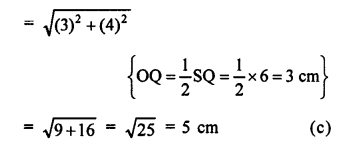Question 3.
Solution:
In the given figure, PT is tangent to the circle with centre O and radius OT = 7 cm, PT = 24 cm
OT is the radius and PT is the tangent OT ⊥ PT
Now, in right ∆OTP,
OP² = OT² + PT²
OP² = (7)² + (24)²
OP² = 49 + 576 = 625 = (25)²
OP = 25 cm (c)

Question 4.
Solution:
Two diameters cannot be parallel. (d)

Question 5.
Solution:
A chord subtends a right angle at its centre
Radius of the circle = 10 cm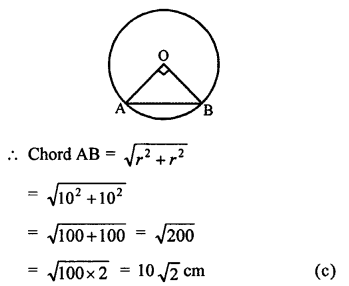Question 6.
Solution:
In the given figure, PT is tangent to the circle with centre O and radius
OT = 6 cm OP = 10 cm
OT is the radius and PT is the tangent
OT ⊥ TB
Now, in right ∆OPT,
OP² = OT² + PT² (Pythagoras Theorem)
⇒ (10)² = (6)² + PT²
⇒ 100 = 36 + PT²
⇒ PT² = 100 – 36 = 64 = (8)².
PT = 8 cm (a)

Question 7.
Solution:
In the given figure, point P is 26 cm away from the centre O of the circle.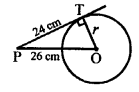Length of tangent PT = 24 cm
In right ∆OPT,
OP² = PT² + OT²
⇒ 26² = 24² + r²
⇒ r² = 26² – 24² = 676 – 576 = 100 = (10)²
r = 10

Question 8.
Solution:
PQ is tangent to the circle with centre O at P. ∆OPQ is an isosceles triangle.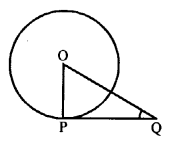∠OQP = ?
∆OPQ is an isosceles triangle
OP = PQ
∠POQ = ∠OQP
But OP is radius and PQ is tangent
OP ⊥ PQ ⇒ ∠OPQ = 90°
∠POQ + ∠OQP = 90°
⇒ ∠POQ = ∠OQP = $$\frac { 90 }{ 2 }$$ = 45°
Hence, ∠OQP = 45° (b)

Question 9.
Solution:
In the given figure, AB and AC are tangents to the circle with centre O such that ∠BAC = 40°, ∠BOC = ?
AB and AC are tangents and OB and OC are radii.
OB ⊥ AB and OC ⊥ AC
⇒ ∠OBA = 90° and ∠OCA = 90°
∠BAC + ∠BOC = 180°
⇒ 40° + ∠BOC = 180°
⇒ ∠BOC = 180° – 40° = 140° (d)

Question 10.
Solution:
A chord AB subtends an angle of 60° at the centre of a circle with centre O.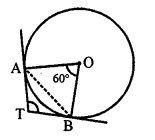TA and TB are tangents drawn to the circle.
Then, ∠ATB = 180° – ∠AOB = 180° – 60° = 120° (d)

Question 11.
Solution:
In the given figure, O is the centre of the two concentric circles of radii 6 cm and 10 cm.
AB is a chord of the outer circle and touches the inner circle at P.
OP = 6 cm, OA = 10 cm
OP is radius and APB is tangent to the inner circle.
OP ⊥ AB and P is the mid point of AB.
In right ∆OPA,
OA² = OP² + AP²
⇒ 10² = 6² + AP²
⇒ 100 = 36 + AP²
⇒ AP²= 100 – 36 = 64 = (8)²
AP = 8 cm
and AB = 2 x AP = 2 x 8 = 16 cm (c)

Question 12.
Solution:
In the given figure, AB and AC are tangents to a circle with centre O and radius 8 cm.
OA = 17 cm.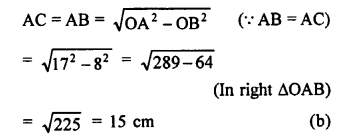Question 13.
Solution:
In the given figure, O is the centre of the circle, AT is tangent, AOC is the diameter and ∠ACB = 50°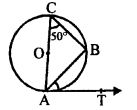We have to find the measure of ∠BAT
AB is chord and AT is the tangent
∠ACB = ∠BAT (Angles in the alternate segment)
= 50° (b)

Question 14.
Solution:
O is the centre of circle, PQ is a chord, PT is tangent.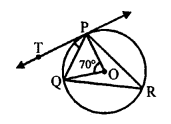∠POQ = 70°, then ∠TPQ = ?
Take a point R on the major segment and join PR and QR
arc PQ subtends ∠POQ at the centre and ∠PRQ at the remaining part of the circle
∠PRQ = $$\frac { 1 }{ 2 }$$ ∠POQ = $$\frac { 1 }{ 2 }$$ x 70° = 35°
But ∠TPQ = ∠PRQ (Angles in the alternate segment)
∠TPQ = 35° (a)

Question 15.
Solution:
In the given figure, AT is the tangent to the circle with centre O and OA is its radius OT = 4 cm, ∠OTA = 30°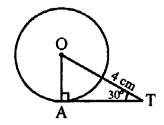Now, we have to find the length of AT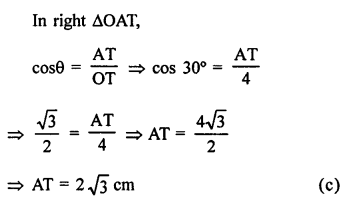Question 16.
Solution:
In the given figure, PA and PB are the two tangents to the circle with centre O, which subtends ∠AOB = 110°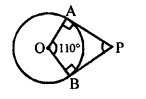Now, we have to find the measure ∠APB
OA and OB are the radii of the circle and AP and BP are the tangents
OA ⊥ AP and OB ⊥ BP
∠A = ∠B = 90°
∠A + ∠B = 90° + 90°= 180°
∠AOB + ∠APB = 180°
⇒ 110° + ∠APB = 180°
⇒ ∠APB = 180° – 110° = 70° (c)

Question 17.
Solution:
In the given figure, in ∆ABC,
BC = ?
AF and AE are the tangents to the circle from A.
AE = AF = 4 cm CE = AC – AE = 11 – 4 = 7 cm
Similarly, CD and CE are tangents
CD = CE = 7 cm
and BF and BD are tangents BD = BF = 3 cm
BC = BD + CD = 3 + 7 = 10 cm (b)

Question 18.
Solution:
In the given figure, ∠AOD = 135°
We know that if a circle is inscribed in a quadrilateral, the opposite sides subtends supplementary angles.
∠AOD + ∠BOC = 180°
135° + ∠BOC = 180°
⇒ ∠BOC = 180° – 135° = 45° (b)

Question 19.
Solution:
In the given figure, PQ is a chord of a circle with centre O and PT is a tangent at P to the circle such that ∠QPT = 50°.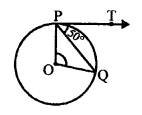Then, we have to find ∠POQ.
PT is the tangent and OP is the radius
OP ⊥ PT ⇒ ∠OPT = 90°
∠OPQ = ∠OPT – ∠QPT = 90° – 50° = 40°
In ∆OPQ,
OP = OQ (radii of the same circle)
∠OPQ = ∠OQP = 40°
and ∠POQ = 180° – (∠OPQ + ∠OQP)
= 180° – (40° + 40°)
= 180°- 80° = 100° (a)

Question 20.
Solution:
In the given figure, PA and PB are two tangents to the circle with centre O.
∠APB = 60° then ∠OAB
Join OB.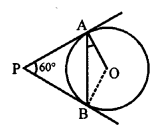∠APB + ∠AOB = 180°
OA is radius and PA is tangent
OA ⊥ AP ⇒ ∠OAP = 90°
PA = PB (Tangents to the circle)
∠PAB = ∠PBA
But, ∠PAB + ∠PBA = 180° – 60° = 120°
∠PAB = ∠PBA = $$\frac { 120 }{ 2 }$$ = 60°
∠OAB = 90° – 60° = 30° (b)

Question 21.
Solution:
Two tangents inclined at an angle of 60° are drawn to a circle of radius 3 cm.
Join OA, OB and OP.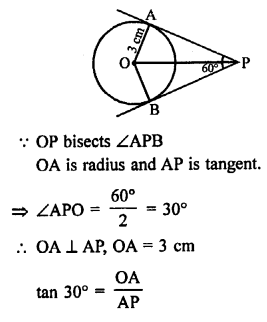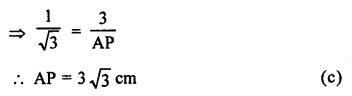Question 22.
Solution:
In the given figure, PQ and PR are tangents drawn from an external point P to a circle with centre A.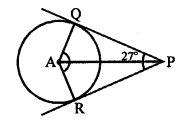∠QPA = 27°, ∠QAR = ?
AP bisects ∠QPR and ∠QPA = 27°
∠QPR = 2 x 27° = 54°
But ∠QPR + ∠QAR = 180° (QARP is a cyclic quadrilateral)
⇒ 54° + ∠QAR = 180°
⇒ ∠QAR = 180° – 54° = 126° (c)

Question 23.
Solution:
In the given figure, PA and PB are two tangents drawn from an external point P to a circle with centre C and radius 4 cm.
PA ⊥ PB, then length of tangent is = ?
Join GA and CB.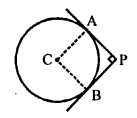CAand CB are radii and PA, PB are tangents to the circle.
CA ⊥ PA and CB ⊥ PB But, ∠APB = 90°
∠ACB = 180° – 90° = 90°
PA = PB tangents of a circle
CAPB is a square
PA = PB = radius = 4 cm (b)

Question 24.
Solution:
In the given figure, PA and PB are tangents to the circle with centre O such that ∠APB = 80°.
Join OP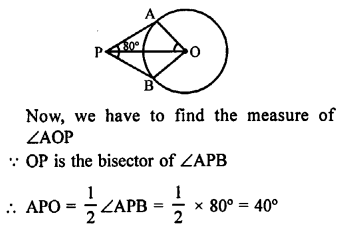Now, in right ∆OAP, ∠A = 90°
∠AOP = 90° – 40° = 50° (b)

Question 25.
Solution:
In the given figure, O is the centre of a circle. AB is the tangent to the circle at point P.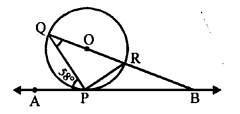∠APQ = 58°, ∠PQB = ?
∠QPR = 90° (Angle in a semi circle)
But, ∠RPB + ∠QPR + ∠APQ = 180° (Angles on one side of a line)
⇒ ∠RPB + 90° + 58° = 180°
⇒ ∠RPB + 148° = 180°
⇒ ∠RPB = 180° – 148° = 32°
∠PQR or ∠PQB = ∠RPB (Angles in the alternate segment)
⇒ ∠PQB = 32° (a)

Question 26.
Solution:
In the given figure, O is the centre of the circle. AB is tangent to the circle at P.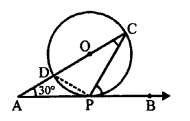∠PAO = 30°
∠CPB + ∠ACP = ?
∠CPD = 90° (Angle in a semi circle)
∠DPA + ∠CPB = 90°
But, ∠DPA = ∠ACP (Angles in alternate segment)
∠CPB + ∠ACP = 90° (b)

Question 27.
Solution:
In the given figure, PQ is the tangent to the circle at A.
∠PAB = 67°, ∠AQB = ?
Join BC.
∠BAC = 90° (Angle in a semi circle)
But, ∠PAB + ∠BAC + ∠CAQ = 180°
⇒ 67° + 90° + ∠CAQ = 180°
⇒ 157° + ∠CAQ = 180°
∠CAQ = 182° – 157° = 23°
∠ACB = ∠PAB (Angles in the alternate segment)
∠ACB = 67°
In ∆ACQ,
Ext. ∠ACB = ∠CAQ + ∠AQC
⇒ 67° = 23° + ∠AQC
⇒ ∠AQC = 67° – 23° = 44°
⇒ ∠AQB = 44° (d)

Question 28.
Solution:
In the given figure, two circles touch each other at C. AB is the common tangent.
∠ACB = ?
Draw a tangent from C which meets AB at P.
PA and PC are tangents to the first circle.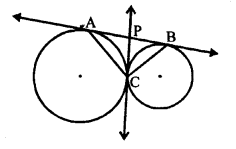PA = PC
∠PAC = ∠PCA …(i)
Similarly, PB = PC
∠PCB = ∠PBC …(ii)
Adding, ∠PAC + ∠PBC = ∠PCA + ∠PCB
⇒ ∠PAC + ∠PBC = ∠ACB
But, ∠PAC + ∠PBC + ∠ACB = 180° (Angles of a triangle)
∠ACB = 90° (c)

Question 29.
Solution:
In the given figure O is the centre of the circle with radius 5 cm P is a point out side the circle and OP = 13 cm
PQ and PR are the tangents to the circle drawn from P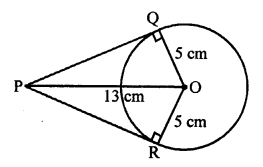We have to find the area of quad. PQOR
OQ is radius and PQ is the tangent
OQ ⊥ QP
In ∆OPQ,
OP² = OQ² + PQ² (Pythagoras Theorem)
⇒ (13)² = (5)² + PQ²
⇒ 169 = 25 + PQ²
⇒ PQ² = 169 – 25 = 144 = (12)²
PQ = 12 cm
PQ = PR = 12 cm
Now, diagonal OP bisects the quad. PQOR into two triangles equal in areas.
Now, area of ∆PQO = $$\frac { 1 }{ 2 }$$ x PQ x OQ
= $$\frac { 1 }{ 2 }$$ x 12 x 5 = 30 cm²
Area of quad. PQOR = 2 x area ∆PQO = 2 x 30 = 60 cm² (a)

Question 30.
Solution:
In the given figure,
PQR is a tangent drawn at Q to the circle with centre O.
AB is a chord parallel to PR such that ∠BQR = 70°
Then, we have to find ∠AQB
Join QO and produce it to AB meeting it at L.
OQ ⊥ PR ⇒ LQ ⊥ PR
QL bisects AB at L
QA = QB
∆QAB is an isosceles triangle
∠LQA = ∠LQB
∠LQA = ∠LQR – ∠BQR = 90° – 70° = 20°
∠AQB = 2 x 20° = 40° (c)

Question 31.
Solution:
Length of a tangent to the circle from an external point = 10 cm
Radius (r) = 5 cm OP = ?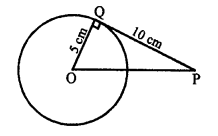OQ is radius and QP is tangent
OQ ⊥ QP
In right ∆OPQ,
OP² = OQ² + QP² (Pythagoras Theorem) = (5)² + (10)² = 25 + 100 = 125
OP = √125 cm (d)

Question 32.
Solution:
In the figure, O is the centre of the circle BOA is its diameter and PT is tangent at P which meets BA produced at T. ∠PBO = 30°.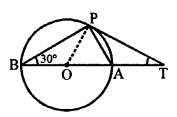We have to find ∠PTA
In ∆BOP,
OB = OP (radii of the same circle)
∠APB = ∠PBO = 30°
But, OP is radius and PT is the tangent
OP ⊥ PT ⇒ ∠OPT = 90°
∠BPT = ∠BPO + ∠OPT = 30° + 90° = 120°
Now, in ∆PBT,
∠BPT + ∠PBA + ∠PTA = 180° (sum of angles of a triangle)
⇒ 120° + 30° + ∠PTA = 180°
⇒ 150° + ∠PTA = 180°
⇒ ∠PTA = 180° – 150° = 30° (b)

Question 33.
Solution:
In the given figure, a circle touches the side DF of a AEDF at H and touches ED and EF on producing at K and M respectively.
EK = 9 cm.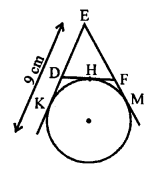Perimeter of ∆EDF.
DH and DK are tangents to the circle.
DH = DK
Similarly, ∠FH = ∠FM and EK = EH = 9 cm
EK = ED + DK ⇒ ED + DH = 9 cm…(i)
Similarly, EH = EF = FH = EF + FM = 9 cm …(ii)
ED + DH + EF + FH = 9 + 9 cm (DH + HF = DF)
ED + DF + FE = 18 cm
Perimeter of ∆EDF = 18 cm (d)

Question 34.
Solution:
In the given figure, PA and PB are two tangents drawn from an external point P which inclined at an angle of 45°.
OA and OB are radii of the circle.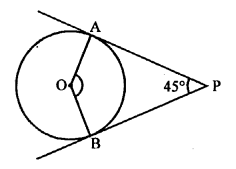To find ∠AOB
∠AOB + ∠APB = 180°
⇒ ∠AOB + 45° = 180°
⇒ ∠AOB = 180° – 45° = 135° (b)

Question 35.
Solution:
In the given figure, O is the centre of the circle PQL and PRM are the tangents from P drawn to the circle meeting it at Q and R respectively ∠SQL = 50°, and ∠SRM = 60°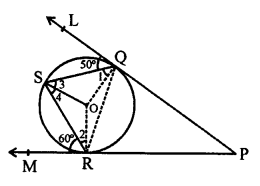Now, we have to find ∠QSR,
Join OQ, OR and OS OQ is radius and QP is tangent
OQ ⊥ QP
Similarly, OR ⊥ RP
∠1 = 90° – 50° = 40° and ∠2 = 90° – 60° = 30°
OS = OQ (radii of the same circle)
∠3 = ∠1 = 40°
Similarly OS = OR
∠2 = ∠4 = 30°
∠QSR = ∠3 + ∠4 = 40° + 30° = 70° (d)

Question 36.
Solution:
In the given figure, a ∆PQR is drawn to inscribe a circle with centre O and radius 6 cm.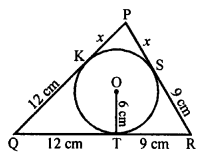QT = 12 cm, TR = 9 cm
Area ∆PQR =189 cm²
PQ = ?
QK = QT = 12 cm
RS = RT = 9 cm
Let PK = PS = x cm
PQ = 12 + x
PR = 9 + x cm
Area of A = $$\frac { 1 }{ 2 }$$ x r x Perimeter of ∆PQR
⇒ 189 = $$\frac { 1 }{ 2 }$$ x 6 x (PQ + QR + RP)
⇒ 189 = 3 (12 + x + 21 + 9 + x)
⇒ 63 = 42 + 2x
⇒ 2x = 63 – 42 = 21
x = 10.5
AB = 10.5 + 12 = 22.5 cm (c)

Question 37.
Solution:
In the given figure, QR is a common tangent to two given circles touching each other externally at point T.
A tangent PT is drawn from T which intersects QR at P.
PT = 3.8 cm, QR = ?
PT and PQ are tangents to the first circle.
PQ = PT …(i)
Similarly, PT and PR tangents to the second circle.
PR = PT …(ii)
From (i) and (ii),
PQ = PR = PT = 3.8 cm
QR = 3.8 + 3.8 = 7.6 cm (d)

Question 38.
Solution:
In the figure, quadrilateral ABCD is circumscribed touches the circle at P, Q, R and S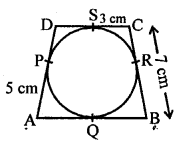AP = 5 cm, BC = 7 cm, CS = 3 cm AB = ?
Tangents drawn from the external point to the circle are equal
AQ = AP = 5 cm
CR = CS = 3 cm
BQ = BR
Now, BR = BC – CR = 7 – 3 = 4 cm
BQ = 4 cm
Now, AB = AQ + BQ = 5 + 4 = 9 cm (a)

Question 39.
Solution:
In the given figure, quad. ABCD is circumscribed touching the circle at P, Q, R and S
AP = 6 cm, BP = 5 cm, CQ = 3 cm and DR = 4 cm.
Now, we have to find the perimeter of the quad. ABCD.
We know that tangents from an external point to the circle are equal.
AP = AS = 6 cm
BP = BQ = 5 cm
CQ = CR = 3 cm
DR = DS = 4 cm
AB = AP + BP = 6 + 5 = 11 cm
BC = BQ + CQ = 5 + 3 = 8 cm
CD = CR + DR = 3 + 4 = 7 cm
and DA = AS + DS = 6 + 4 = 10 cm
= AB + BC + CD + DA
= (11 + 8 + 7 + 10) cm
= 36 cm (c)

Question 40.
Solution:
In the given figure, O is the centre of the circle, AB is chord and AT is the tangent at A.
∠AOB = 100°, ∠BAT = ?
Take a point P on the major segment of the circle and join AP and BP.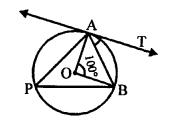Arc AB subtends ∠AOB at the centre and ∠APB at the remaining part of the circle.
∠APB = $$\frac { 1 }{ 2 }$$ ∠AOB = $$\frac { 1 }{ 2 }$$ x 100° = 50°
Now, ∠BAT = ∠APB (Angles in the alternate segment)
∠BAT = 50° (b)

Question 41.
Solution:
In a right ∆ABC, right angled at B
BC = 12 cm, AB = 5 cm
A circle is inscribed in it touching its sides at P, Q and R.
Join OP, OQ and OR.
AC² = AB² + BC² (Pythagoras Theorem)
= 5² + 12² = 25 + 144 = 169 = (13)²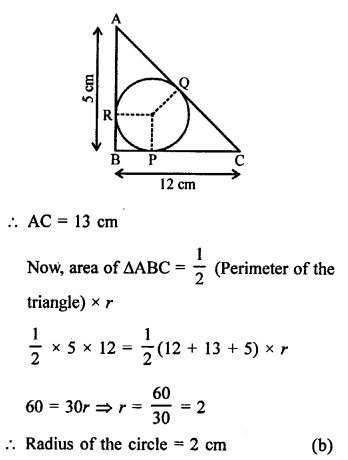Question 42.
Solution:
In the given figure, a circle is inscribed in a quadrilateral ABCD touching its sides, AB, BC, CD and DA at P, Q, R and S respectively
BC = 38 cm, PB = 27 cm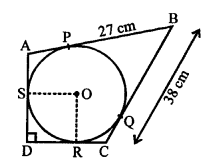Length of CD = ?
Join OR and OS
BP and BQ are tangents to the circle
BQ = BP = 27 cm
BC = 38 cm
QC = 38 – 27 = 11 cm
CQ and CR are the tangents to the circle
CR = CQ = 11 cm
DR and DS are the tangents to the circle
DR = DS
Similarly, OR ⊥ DC
OSDR is a square whose each side is equal to the radius = 10 cm
DR = DS = 10 cm
CD = CR + DR = 11 + 10 = 21 cm (d)

Question 43.
Solution:
In the given figure, ∆ABC is a right angled triangle, right angle at ∠B.
BC = 6 cm, AB = 8 cm
A circle with centre O is inscribed inside the triangle ABC
OP ⊥ AB and OQ ⊥ BC and OR ⊥ AC
OP = OQ = OR = x cm
OPBQ is a square
In right ∆ABC,
AC² = AB² + BC² (Pythagoras Theorem)
= (8)² + (6)² = 64 + 36 = 100 = (10)²
AC = 10 cm
Tangents drawn from the external point to the circle are equal
BP = BQ = x
CQ = CR = 6 – x
AP = AR = 8 – x
AR + CR = AC
⇒ 8 – x + 6 – x = 10
⇒ 14 – 2x = 10
⇒ 2x = 14 – 10 = 4
x = 2
Hence r = 2cm (a)

Question 44.
Solution:
A quadrilateral ABCD is circumscribed to a circle with centre O.
AB = 6 cm, BC = 7 cm, CD = 4 cm, AD = 7 cm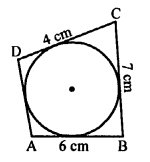ABCD circumscribed to a circle.
AB + CD = BC + AD
⇒ 6 + 4 = 7 + AD
⇒ 10 = 7 + AD
AD = 10 – 7 = 3 cm (a)

Question 45.
Solution:
In the given figure, PA and PB are the tangents to the circle with centre O from P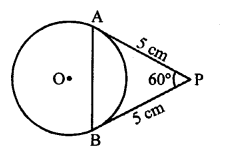PA = 5 cm, ∠APB = 60°
PA = PB = 5 cm
In ∆APB, ∠P = 60° and PA = PB
PAB is an equilateral triangle
AB = AP = BP = 5 cm (b)

Question 46.
Solution:
In the given figure, DE and DF are tangents to the circle from an external point D.
A is the centre of the circle.
DF = 5 cm and DE ⊥ DF, radius of the circle = 3
Join EA and FA.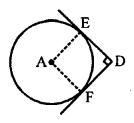AE and AF are the radius of the circle and DE and BF are the tangents.
AE ⊥ DE and AF ⊥ DF
∠EAF = 180° – ∠EDF = 180° – 90° = 90°
AEDF is a square.
AE = 5 cm
Radius of the circle = 5 cm (c)

Question 47.
Solution:
In the given figure, three circles with centre A, B and C are drawn touching each other externally
AB = 5 cm, BC = 7 cm and CA = 6 cm
Let r1, r2, r3 be the radii of three circles respectively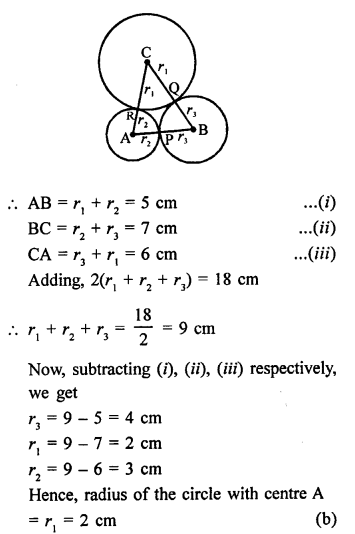Question 48.
Solution:
In the given figure, AP, AQ and BC are tangents to the circle.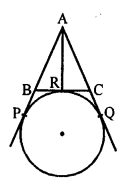AB = 5 cm, AC = 6 cm, BC = 4 cm
Length of AP = ?
BP and BR are the tangents to the circle.
BP = BR
Similarly, CR and CQ are tangents
CR = CQ
S and AP and AQ are tangents
AP = AQ
AP = AB + BP = AB + BR
AQ = AC + CQ = AC + CR
AP + AQ = AB + BR + AC + CR = AB + BR + CR + AC
AP + AP = AB + BC + AC
2AP = 5 + 4 + 6 = 15 cm
AP = $$\frac { 15 }{ 2 }$$ = 7.5 cm (d)

Question 49.
Solution:
In the given figure, O is the centre of two concentric circles of radii 5 cm and 3 cm respectively.
From external point P, PA and PB are tangents are drawn to the external circle and internal circle respectively
PA = 12 cm, PB = ?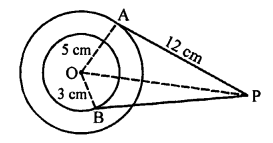OA and OB are the radii
OA ⊥ AP and OB ⊥ BP
Now, in right ∆OAP,
OP² = OA² + AP² (Pythagoras Theorem)
= (5)² + (12)²
= 25 + 144 = 169 = (13)²
OP = 13 cm
and in right ∆OBP,
OP² = OB² + BP²
(13)² = (3)² + BP²
⇒ 169 = 9 + BP²
⇒ PB² = 169 – 9 = 160
PB = √160 = √(16 x 10) = 4√10 cm (c)

True/False Type
Question 50.
Solution:
(a) It is true that no tangent can be drawn from a point inside the circle.
(b) It is true, that one and only one tangent can be drawn from a point on the circle.
(c) True. If a point P is outside the circle, two tangents can be drawn to the circle.
(d) No, only two parallel tangents can be drawn which are parallel to a given line. (d)

Question 51.
Solution:
(a) It is true as a tangent intersects (touches) the circle exactly at one point.
(b) It is true that common point to the circle where the tangent touches the circle is called point of contact.
(c) It is true that the radius through the point of contact of a tangent is perpendicular to it.
(d) False as a straight line can meet at the most two points. (d)

Question 52.
Solution:
(a) It is true, that a secant is a line which intersects the circle at two points.
(b) It is true, as a tangent intersects the circle at only one point.
(c) It is true that the point at which a tangent touches the circle is called a point of contact.
(d) It is false, as no tangent can be drawn from a point in side the circle.

Assertion and Reason Type
Each question consists of two statements, namely, Assertion (A) and Reason (R).
For selecting the correct answer, use the following code :
(a) Both Assertion (A) and Reason (R) are true and Reason (R) is a correct explanation of Assertion (A).
(b) Both Assertion (A) and Reason (R) are true but Reason (R) is not a correct explanation of Assertion (A).
(c) Assertion (A) is true and Reason (R) is false.
(d) Assertion (A) is false and Reason (R) is true.
Question 53.
Solution:
In Assertion (A):
In right ∆OPQ, OP ⊥ PQ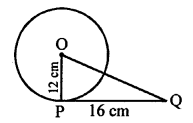OQ² = OP² + PQ² = (12)² + (16)² = 144 + 256 = 400 = (20)²
OQ = 20 cm, which is true
In Reason (R):
It is also with respect to (A) (a)

Question 54.
Solution:
Assertion (A):
The statement is true
In Reason (R):
It is also true but not with respect to (A) (b)

Question 55.
Solution:
In Assertion (A):
In the figure, ABCD is a quad, which is circumscribed a given circle.
Sum of opposite sides are equal
AB + CD = BC + AD
It is not true that AB + BC = AD + DC
In Reason (R):
It is true but not with respect to Assertion (A) (d)

Hope given RS Aggarwal Solutions Class 10 Chapter 12 Circles MCQS are helpful to complete your math homework.

If you have any doubts, please comment below. Learn Insta try to provide online math tutoring for you.